## Calculate Stock Rate of Return

This post will discuss that how to calculate the rate of return for the share of stock in Excel. And to calculate the return for a share of stock, you need to know the starting price, ending price and dividends paid and the duration for which the stock was held.

## Calculate Stock Return

You can try to calculate the rate of return by manually, or you use an Excel formula to achieve the result. The best way to calculate your rate of return is to use the EXCEL XIRR function, and this function is a financial function in Excel.

For example, you bought stock “IBM” in 2015, 100 shares for \$164 each. And IBM pays dividends quarterly. And the ending price is \$180.  And the below is table of dividends, starting price and ending price:

 Date price/Dividend 1-Jan-15 -\$164.00 30-Apr-15 \$1.50 30-Aug-15 \$2.50 30-Dec-15 \$1.80 1-Jan-16 \$180.00

So you can use the below formula to calculate the rate of return for your stock:

`=XIRR(B2:B6,A2:A6)`

#1 type the above formula in the formula box of cell C2, press enter key. You will get the rate of return of the stock you bought.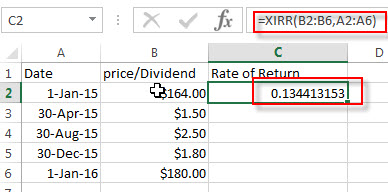#2 select the cell that contain rate of return (C2), then right-click on it, select Format Cell…  from the drop-down list.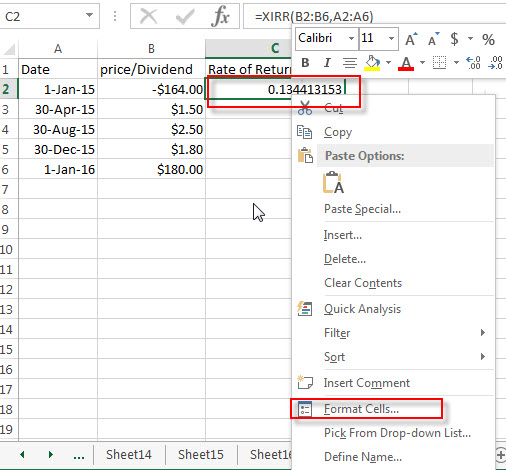#3 click Percentage under Category section. Click OK button.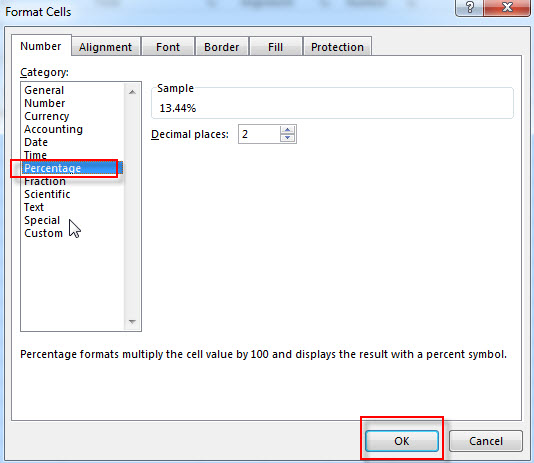#4 now you can see that the rate of return for your stock is shown as a percentage.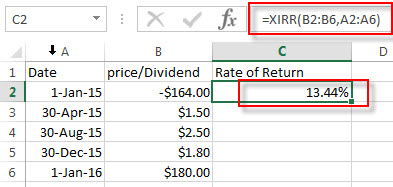### Related Functions

• Excel XIRR Function
The Excel XIRR function returns the internal rate of return for a series of cash flows that is not necessarily periodic.The syntax of the XIRR function is as below:= XIRR (values, dates, [guess])…

## Excel XIRR Function

This post will guide you how to use Excel XIRR function with syntax and examples in Microsoft excel.

### Description

The Excel XIRR function returns the internal rate of return for a series of cash flows that is not necessarily periodic. And if you want to get the internal rate of return for a series of periodic cash flows, you can use the IRR function in Excel.

The XIRR function is a build-in function in Microsoft Excel and it is categorized as a Financial Function.

The XIRR function is available in Excel 2016, Excel 2013, Excel 2010, Excel 2007, Excel 2011 for Mac.

### Syntax

The syntax of the XIRR function is as below:

`= XIRR (values, dates, [guess])`

Where the XIRR function arguments are:

• Values -This is a required argument. An array or cell reference that contain numbers for which you want to calculate the internal rate of return. And the values must include at least one positive value and one negative value.
• Dates -This is a required argument. A series of dates that corresponds to the cash flow payments.
• Guess – This is an optional argument. An initial guess at the internal rate of return. If it is omitted, and it will be set as 0.1 or 10%.

Note:

• The values arguments must contain at least one positive value and one negative cash flow value, or the XIRR function will return the #NUM! Error.
• If you provide an invalid date in the date argument, the XIRR function will return the #NUM! Error.

### Excel XIRR Function Examples

The below examples will show you how to use Excel XIRR Function to calculate the internal rate of return for a series of cash flows.

#1 to get the internal rate of return, using the following formula:

`=XIRR(A2:A5,B2:B5)`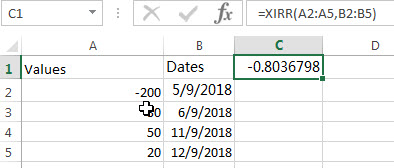### Related Functions

• Excel IRR Function
The Excel IRR function returns the internal rate of return for a series of cash flows and the cash flows must be occurred at regular intervals (monthly or annually).The syntax of the IRR function is as below:=IRR(values, [guess])…

### Related Examples

• Calculate Stock Rate of Return
You can try to calculate the rate of return by manually, or you use an Excel formula to achieve the result. The best way to calculate your rate of return is to use the EXCEL XIRR function,…